﻿ Comp310: Lec15

# Lec15:  Collisions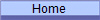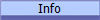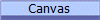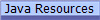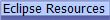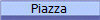See the demo of the command-dispatching Ballworld with inter-ball interactions

The discussion below should be viewed in terms of two separate processes:

1. A criteria for interaction, e.g. overlapping radii, same/different color, aligned with velocity, etc.
2. An interaction behavior, e.g. elastic bouncing, spawning, eating, etc.

In the end, you should modify the code below such that these two separate processes are clearly expressed in your architecture, which enables you to separately vary the concrete implementations of these processes.

## Collision Handling

Basic physics of elastic collisions of two spherical objects of different mass: 2-Body Elastic Collisions

Make sure that the other ball does not have infinite mass (mass = Double.POSITIVE_INFINITY)!   (Note:  balls with infinite mass and non-zero velocities technically have infinite kinetic energy, so they tend to continuously add energy to the system, which quickly causes problems.)   We will use a mass here that is proportional to the area of the ball (square of the radius), so we will not encounter this issue here, but I mention it if you wish to separate the mass as an independent quantity and thus create things like infinitely heavy "rock" balls.

CollideStrategy is a strategy that adds elastic collisions to a ball. It provides a number of utility methods that by encapsulating many of the more mathematical calculations,  help the main calculations focus on what they are fundamentally trying to achieve.  Read the documentation below very carefully!

Basic recipe for a collision command:

1. Send a command out to all the other balls to see if anyone has collided with the context ball and to process that collision accordingly.    How will this command, which is being executed by another ball "remember" who the sending ball is and thus who to compare against?
2. Make sure that the other ball is not yourself!    Remember that the dispatcher sends the command to everybody!
3. Are the two balls within collision distance?
• If so, then proceed to calculate the effect of the collision on each other.
1. Calculate the reduced mass of the two-ball system using the square of the radius as the mass of the ball (mass is proportional to the size of the ball).
2. Use the reduced mass and other parameters to calculate the impulse of the collision.   The position of the source ball will be "nudged" out of collision distance during this calculation.
3. Update the velocities of each ball  by taking the impulse divided by the mass (square of the radius)  and call its post-collision interaction behavior (the interactWith method of the Ball).   Note that the same method can be used to update either the source or target balls simply by switching the parameters and negating the impulse.    Careful here!  This is simpler than it looks!   Use all the resources available to you!
• Otherwise, the command should end.   The dispatcher will send the command to the next ball to be processed.

Here is most of code for CollideStrategy showing the utility methods that are used:

```	public class CollideStrategy extends  AUpdateStrategy {

@Override
public void updateState(final Ball context, Dispatcher dispatcher) {
// TODO: To be completed by student
}

/**
* Returns the reduced mass of the two balls (m1*m2)/(m1+m2) Gives correct
* result if one of the balls has infinite mass.
*
* @param mSource
*            Mass of the source ball
* @param mTarget
*            Mass of the target ball
*/
protected double reducedMass(double mSource, double mTarget) {
if (mSource == Double.POSITIVE_INFINITY)
return mTarget;
if (mTarget == Double.POSITIVE_INFINITY)
return mSource;
else
return (mSource * mTarget) / (mSource + mTarget);
}

/**
* The amount to add to the separation distance to insure that the two balls
* are beyond collision distance
*/
private double Nudge = 1.1;

/**
* Calculates the impulse (change in momentum) of the collision in the
* direction from the source to the target This method calculates the
* impulse on the source ball. The impulse on the target ball is the
* negative of the result. Also moves source ball out of collision range
* along normal direction. The change in velocity of the source ball is the
* impulse divided by the source's mass The change in velocity of the target
* ball is the negative of the impulse divided by the target's mass
*
* Operational note: Even though theoretically, the difference in velocities
* of two balls should be co-linear with the normal line between them, the
* discrete nature of animations means that the point where collision is
* detected may not be at the same point as the theoretical contact point.
* This method calculates the rebound directions as if the two balls were
* _at_the_point_of_collision_detection_. This may give slightly different
* rebound direction than one would calculate if they contacted at the
* theoretical point given by their actual radii.
*
* @param lSource
*            Location of the source ball
* @param vSource
*            Velocity of the source ball
* @param lTarget
*            Location of the target ball
* @param vTarget
*            Velocity of the target ball
* @param reducedMass
*            Reduced mass of the two balls
* @param distance
*            Distance between the two balls.
* @param deltaR
*            The minimum allowed separation(sum of the ball radii) minus the actual separation(distance between ball centers). Should be a
*            positive value.  This is the amount of overlap of the balls as measured along the line between their centers.
* @return
*/
protected Point2D.Double impulse(Point lSource, Point vSource,
Point lTarget, Point vTarget, double reducedMass, double distance,
double deltaR) {
// Calculate the normal vector, from source to target
double nx = ((double) (lTarget.x - lSource.x)) / distance;
double ny = ((double) (lTarget.y - lSource.y)) / distance;

// delta velocity (speed, actually) in normal direction, source to
// target
double dvn = (vTarget.x - vSource.x) * nx + (vTarget.y - vSource.y)
* ny;

// move the source ball beyond collision range of the target ball, along
// the normal direction.
lSource.translate((int) Math.ceil(-nx * (Nudge * deltaR)),
(int) Math.ceil(-ny * (Nudge * deltaR)));

return new Point2D.Double(2.0 * reducedMass * dvn * nx, 2.0
* reducedMass * dvn * ny);
}

/**
* Updates the velocity of the source ball, given an impulse, then uses the
* context's interactWith method to determine the post collision behavior, from the context
* ball's perspective. The change in velocity is the impulse divided by the (source) ball's mass. To change
* the velocity of the target ball, switch the source and target input
* parameters and negate the impulse values.   This will also run the post collision behavior from
* the other perspective.
*
* @param context
*            The ball to update
* @param target
*            The ball being collided with
* @param impX
*            x-coordinate of the impulse
* @param impY
*            y-coordinate of the impulse
*/
protected void updateCollision(Ball context, Ball target, double impX,
double impY, Dispatcher dispatcher) {

context.getVelocity().translate((int) Math.round(impX / mContext),(int) Math.round(impY / mContext));
context.interactWith(target, dispatcher);
}

}
```

Nudging:

Because of the discrete nature of the movement of the balls and because the Ballworld system uses a single-pass updating mechanism (vs. a two-pass system where updating behaviors are calculated on the first pass and executed on a second pass),  the balls may not be in the exact positions that one would expect them to be when they contact each other.

It is non-trivial to calculate the exact theoretical contact position of the two balls, so the CollideStrategy class pretends that the radius of the balls is exactly the size such that the initial contact between the balls occurrs right at their current location.

In such, all calculations are based off of the "normal vector" which runs from the center of the source ball, through the point of contact between the balls, to the center of the target ball.  This is the line that is normal to the plane of contact between the two balls, if their radii where exactly the size such they just touched at their current locations.   A round or spherical surface can only exert a force along the normal to its tangential planes.

Theoretically, this normal line would be co-linear with the difference in velocities between the two balls (their relative motion, which is used to calculate the impulse), but in actuality, the two are not co-linear.

The shortest distance to move the source ball to get it out of collision distance with the target ball is along the normal direction.   A "nudge" factor is introduced to insure that that the source ball is far enough away to not "double collide" with the target ball.   (Why don't you move the target ball?)

Since the above "nudging" borders on a "hack" because it does not fit into any reasonable abstraction of the collision process, there are bound to be lingering errors.   For instance, it is normal to see strange collision behaviors that look like multiple collisions, as if the nudging process didn't work, when a small, fast-moving ball collides with a large, slow-moving ball.    Can you figure out why this would happen?  Hint: consider the discrete nature of the Ballworld simulation process and possible collision scenarios for a small, fast ball and a large, slow one.

For those with an interest in a much more rigorous treatment of where the ball should be placed, please read the following derivation and code for the elastic rebounding between balls of unequal radii and mass.

Interaction Strategies

In trying to get a large system to run, it is tempting to simply add methods and classes in to "get it to work".   But we must always return to the central driving force: our software as a model of our system.

The post-collision behavior is fundamentally different than the behavior as defined by an IUpdateStrategy.    An IUpdateStrategy defines the behavior of a single ball, in isolation.    On the other hand, the post-collision behavior is necessarily the behavior of 2 balls in relationship to each other.    This overall behavior can be expressed as two single behaviors, one for each ball.  Note that each single behavior depends on both balls and that they are not necessarily the same or mirror images of each other.  For instance, one ball may adjust its velocity to point in parallel with the other ball's, but the other ball's behavior may be to kill the first ball.  We see the two individual interaction behaviors being invoked in Step 2.c above.

Thus, an IInteractStrategy has been added to the Ball class.   In addition to the usual getter and setter, there is a method, public void interactWith(Ball target, Dispatcher disp) that is used to invoke the ball's interaction strategy.

```/**
* Strategy that defines a directed interaction between two balls where the balls are NOT
* equivalently processed.
*
* @author swong
*
*/
public interface IInteractStrategy {

/**
* Performs a directed interaction between the context ball and the target Ball from the
* perspective of the context Ball.
* @param context  The Ball from whose perspective the interaction
* processing takes place.
* @param target  The Ball that is the "other ball" in the perspective of this processing.
* @param disp  The Dispatcher that is to be used if desired.
*/
public void interact(Ball context, Ball target, Dispatcher disp);

/**
* Null strategy with no-op behavior.
*/
public static final IInteractStrategy NULL_STRATEGY = (context, target, disp)->{};
}```

Note that the entire collision interaction can be done as an interaction strategy!    The above code is NOT gospel!!

Strategy Initialization

Just like paint strategies, update strategies may need to be initialized before they are ready to be used.   Sometimes, all a strategy does is to initialize, installing or modifying parts of the ball that will change it's behavior later.   For instance, the initialization may simply install an interaction strategy into the context ball.

Remember to initialize the strategy, even when it comes in via a constructor, i.e. always delegate the setting of the strategy to a setter method which is where the initialization should take place.

Consider a "kill" strategy  that deletes other balls if they collide.   This KillStrategy relies on another IUpdateStrategy to detect the collision and process any rebounding.    The KillStrategy only inserts an IInteractionStrategy that does the "killing" part of the interaction process.

```public class KillStrategy implements  IUpdateStrategy {

public void init(Ball context) {
context.setInteractStrategy(new MultiInteractStrategy(context.getInteractStrategy(), new IInteractStrategy(){
@Override
public void interact(Ball context, Ball target, Dispatcher disp) {
disp.deleteObserver(target);
}

}));
}
@Override
public void updateState(Ball context, Dispatcher disp){
// No-op
}
}

// Or, using lambda expressions:
public class KillStrategy implements  IUpdateStrategy {

public void init(Ball context) {
context.setInteractStrategy(new MultiInteractStrategy(context.getInteractStrategy(), (contextBall, targetBall, disp) -> disp.deleteObserver(targetBall)));
}

@Override
public void updateState(Ball context, Dispatcher dispatcher) {
// No-op
}

}

```

Notice that no assumption is made about whether or not the ball had an existing interaction strategy.

### The Dynamic Nature of Code

Code and design are dynamic living things, ever changing to meet new changing requirements as well as simply a changing outlook and understanding of the problem.   Looking back at the above code, could the entire collision process be considered simply an interaction detection plus interaction behaviors?   That is, could part or all of the code past the actual collision detection be moved to an interaction strategy?   If so, how would you handle the shared portion of the calculation, e.g. the impulse calculation, which is invariant for both balls?

But if we break elastic collisions into a  two-part notion of a detection algorithm followed by interaction strategies, could this architecture be used for more than contact-based interaction?   How about interaction at a distance or other relative location, or interaction by type of ball?       What does this all lead to?